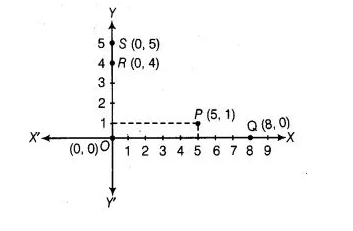# If P(5,1), Q(8, 0), R(0, 4), S(0, 5) and O(0, 0) are plotted on the graph paper,`
Question:

If P(5,1), Q(8, 0), R(0, 4), S(0, 5) and O(0, 0) are plotted on the graph paper, then the points) on the X-axis is/are

(a) P and R

(b) R and S

(c) Only Q

(d) Q and O

Solution:

(d) We know that, a point lies on X-axis, if its y-coordinate is zero. So, on plotting the given points on graph paper, we get Q and O lie on the X-axis.